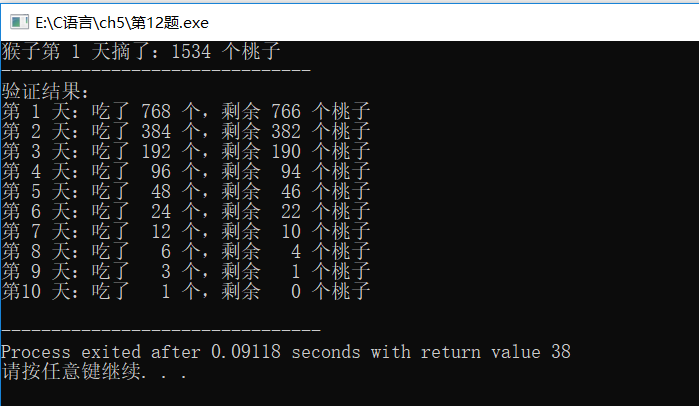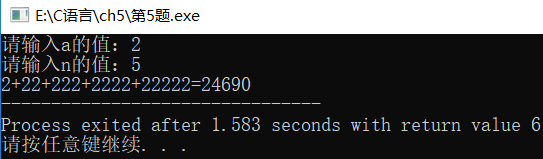# C 代码：

``````#include <stdio.h>

int main() {
//      row：表示 行
// column：表示 列
int row,column;

//设置 <第0行第0列> 值为1
//设置 <第1行第0列、第1列> 值为1
int arr={{1}, {1,1}};

for(row=2; row<10; row++){
arr[row] = 1;//设置第 row 行第 0 列的值为 0
for(column=1; column<row; column++){
//该值 = 上一行<该列-1> + 上一行<该列> 的值
//例如：row=2, column=1，则 arr 的值为
//arr[2-1][1-1]+arr[2-1]
arr[row][column] = arr[row-1][column-1]+arr[row-1][column];
}
arr[row][column] = 1;//设置第 row 行第 column 列的值为 1
}

printf("输出<10行>杨辉三角：\n");
for(row=0; row<10; row++){
for(column=0; column<10; column++){
if(arr[row][column] != 0){//输出值不为0的数
printf("%4d", arr[row][column]);
}
}
printf("\n");
}
}``````

# 解题思路

1.假设 桃子 总共有 `x 个`，则可以列出式子：

y1：第1天剩下的桃子数量
y2：第2天剩下的桃子数量
...
y9：第9天剩下的桃子数量
``````y1 = x/2 - 1
y2 = y1/2 - 1
y3 = y2/2 - 1
y4 = y3/2 - 1
y5 = y4/2 - 1
y6 = y5/2 - 1
y7 = y6/2 - 1
y8 = y7/2 - 1
y9 = y8/2 - 1``````

2.根据题目得知，第9天还剩一个桃子，故我们可解方程得出：

``````因为 y9 = 1

y8 = 1+1*2
y8 = 4

# C 代码

``````#include <stdio.h>

int main() {
int days=1, peach=1, eat;

do{
peach = (peach+1)*2;
days++;
}while(days<10);
printf("猴子第 1 天摘了：%d 个桃子", peach);

printf("\n-------------------------------\n");
printf("验证结果：\n");

days = 1;
do{
eat = peach/2+1;//吃了多少个桃子
peach -= eat;//桃子的剩余数量
printf("第%2d 天：吃了 %3d 个，剩余 %3d 个桃子\n",days,eat,peach);
days++;
}while(days<=10);

}``````

# 运行结果

``````猴子第 1 天摘了：1534 个桃子
-------------------------------# C 代码

``````#include <stdio.h>

int main() {
//molecule：分子
//denominator：分母
double i, sum, molecule, denominator, tmp;

molecule = 2;//初始分子值
denominator = 1;//初始分母值
for(i=1; i<=20; i++){
sum += molecule/denominator;

printf("第 %.0f  项：", i);
printf("%.0f/%.0f\n", molecule, denominator);

tmp = molecule;//用 tmp 暂存 分子
molecule = molecule+denominator;//下一个分子 = 分子+分母
denominator = tmp;//下一个分母 = 前一项得分子
}

printf("\n这个数列的前20项之和为：%f", sum);
}``````

# 运行结果：

``````第 1 项：2/1

# C 代码实现：

``````#include <stdio.h>
#include <math.h>

int main() {
int S,a,n,t;
int i,j;

printf("请输入a的值：");
scanf("%d", &a);

printf("请输入n的值：");
scanf("%d", &n);

if(n<=1){
printf("n 的值必须大于 1");
return 0;//退出程序
}

S = a;
//printf("第1次：%d\n", a);
printf("%d", a);

for(i=1; i<n; i++){
t = 0;

for(j=i; j>=0; j--){
t = t+a*pow(10, j);
}

printf("+%d", t);
//printf("第%d次：%d\n", i+1, t);
S = S+t;
}

printf("=%d", S);

return 0;
}``````

# 运行结果：

``````请输入a的值：2

2+22+222+2222+22222=24690``````## C 语言 | 选择结构程序设计, 第12题

10m，塔以外无建筑物。今输入任何一点的坐标，求该点的建筑高度（塔外的高度为 0m）

# C 语言代码：

``````#include <stdio.h>
#include <math.h>

int main() {
double x,y,d1,d2,d3,d4;

printf("请输入坐标：");
scanf("%lf %lf", &x, &y);

d1 = sqrt(pow(x-2, 2)+pow(y-2, 2));//第一象限    2, 2
d2 = sqrt(pow(x+2, 2)+pow(y-2, 2));//第二象限   -2, 2
d3 = sqrt(pow(x+2, 2)+pow(y+2, 2));//第三象限   -2,-2
d4 = sqrt(pow(x-2, 2)+pow(y+2, 2));//第四象限    2,-2

if(d1<=1 || d2<=1 || d3<=1 || d4<=1) {
printf("该点的建筑高度是 10m");
} else {
printf("该点的建筑高度是 0m");
}

return 0;
}``````

# 运行结果：

``````请输入坐标：1.5 2.3
x=1.500000      y=2.300000

--------------------------------
Process exited after 7.869 seconds with return value 0